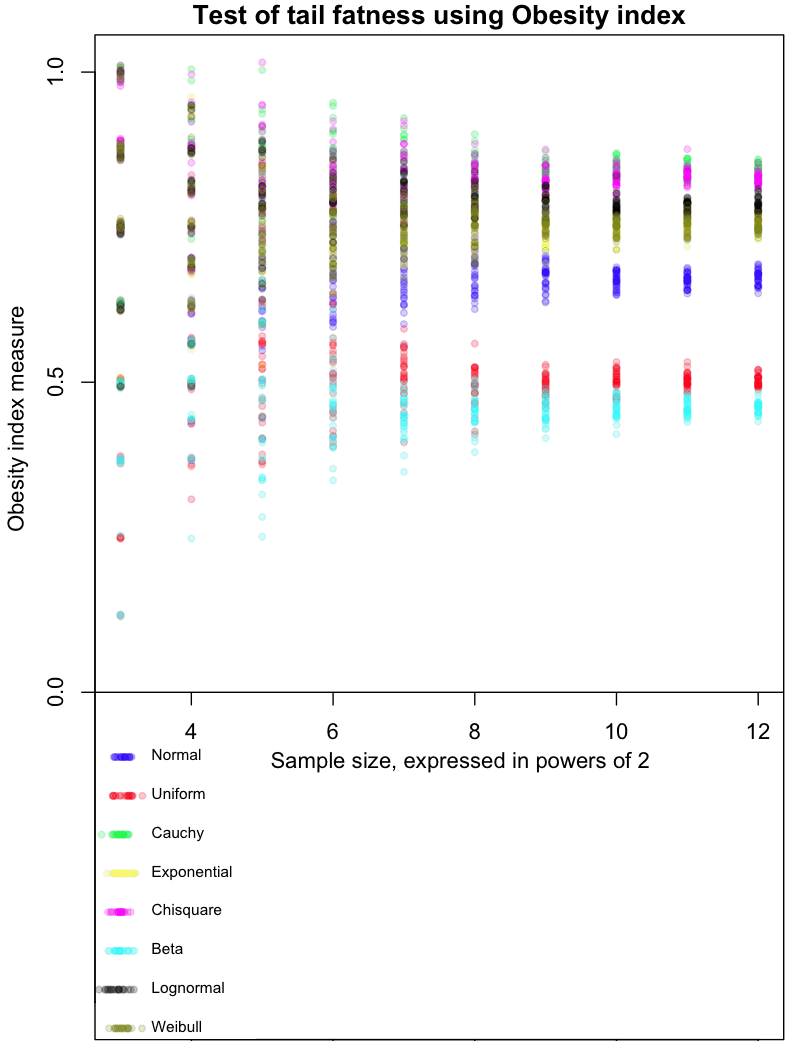[This article was first published on Probability and statistics blog » r, and kindly contributed to R-bloggers]. (You can report issue about the content on this page here)
Want to share your content on R-bloggers? click here if you have a blog, or here if you don't.In my never ending quest to find the perfect measure of tail fatness, I ran across this recent paper by Cooke, Nieboer, and Misiewicz. They created a measure called the “Obesity index.” Here’s how it works:

• Step 1: Sample four times from a distribution. The sample points should be independent and identically distributed (did your mind just say “IID”?)
• Step 2: Sort the points from lowest to highest (that’s right, order statistics)
• Step 3: Test whether the sum of the smallest and greatest number is larger than the sum of the two middle.

The Obesity index is the probability that the sum of these end points is larger than the sum of the middle numbers. In mathy symbols:

but not to translations:$Ob(X) \neq Ob(X+c)$

This could be a bug or a feature, depending on what you want to use the index for.

Extra special karma points to the first person who comes up with a distribution whose Obesity index is between the Uniform and Normal, and that isn’t a variant of one I already tested.

Here’s the code:

# Code by Matt Asher for StatisticsBlog.com
# Feel free to redistribute, but please keep this notice

# Create random varaibles from the function named in the string
generateFromList = function(n, dist, ...) {
match.fun(paste('r', dist, sep=''))(n, ...)
}

# Powers of 2 for testAt
testAt = 3:12
testAtSeq = 2^testAt
testsPerLevel = 30

distros = c()

distros = 'generateFromList(4,"norm")'
distros = 'generateFromList(4,"unif")'
distros = 'generateFromList(4,"cauchy")'
distros = 'generateFromList(4,"exp")'
distros = 'generateFromList(4,"chisq",1)'
distros = 'generateFromList(4,"beta",.01,.01)'
distros = 'generateFromList(4,"lnorm")'
distros = 'generateFromList(4,"weibull",1,1)'

# Gotta be a better way to do this.
dWords = c("Normal", "Uniform", "Cauchy", "Exponential", "Chisquare", "Beta", "Lognormal", "Weibull")

par(mar=c(4,5,1.5,.5))
plot(0,0,col="white",xlim=c(min(testAt),max(testAt)), ylim=c(-.5,1), xlab="Sample size, expressed in powers of 2", ylab="Obesity index measure", main="Test of tail fatness using Obesity index")

abline(h=0)

colorList = list()
colorList[]=rgb(0,0,1,.2)
colorList[]=rgb(1,0,0,.2)
colorList[]=rgb(0,1,0,.2)
colorList[]=rgb(1,1,0,.2)
colorList[]=rgb(1,0,1,.2)
colorList[]=rgb(0,1,1,.2)
colorList[]=rgb(0,0,0,.2)
colorList[]=rgb(.5,.5,0,.2)

# Create the legend
for(d in 1:length(distros)) {
x = abs(rnorm(20,min(testAt),.1))
y = rep(-d/16,20)
points(x, y, col=colorList[[d]], pch=20)
text(min(testAt)+.25, y, dWords[d], cex=.7, pos=4)

}

dCounter = 1
for(d in 1:length(distros)) {
for(l in testAtSeq) {
for(i in 1:testsPerLevel) {
count = 0
for(m in 1:l) {

# Get the estimate at that level, plot it testsPerLevel times
x = sort(abs(eval(parse( text=distros[dCounter] ))))
if ( (x+x)>(x+x) ) {
count = count + 1
}
}

# Tiny bit of scatter added
ratio = count/l
points(log(l, base=2), ( ratio+rnorm(1,0,ratio/100)), col=colorList[[dCounter]], pch=20)
}
}

dCounter = dCounter + 1
}

To leave a comment for the author, please follow the link and comment on their blog: Probability and statistics blog » r.

R-bloggers.com offers daily e-mail updates about R news and tutorials about learning R and many other topics. Click here if you're looking to post or find an R/data-science job.
Want to share your content on R-bloggers? click here if you have a blog, or here if you don't.

# Never miss an update! Subscribe to R-bloggers to receive e-mails with the latest R posts.(You will not see this message again.)

Click here to close (This popup will not appear again)